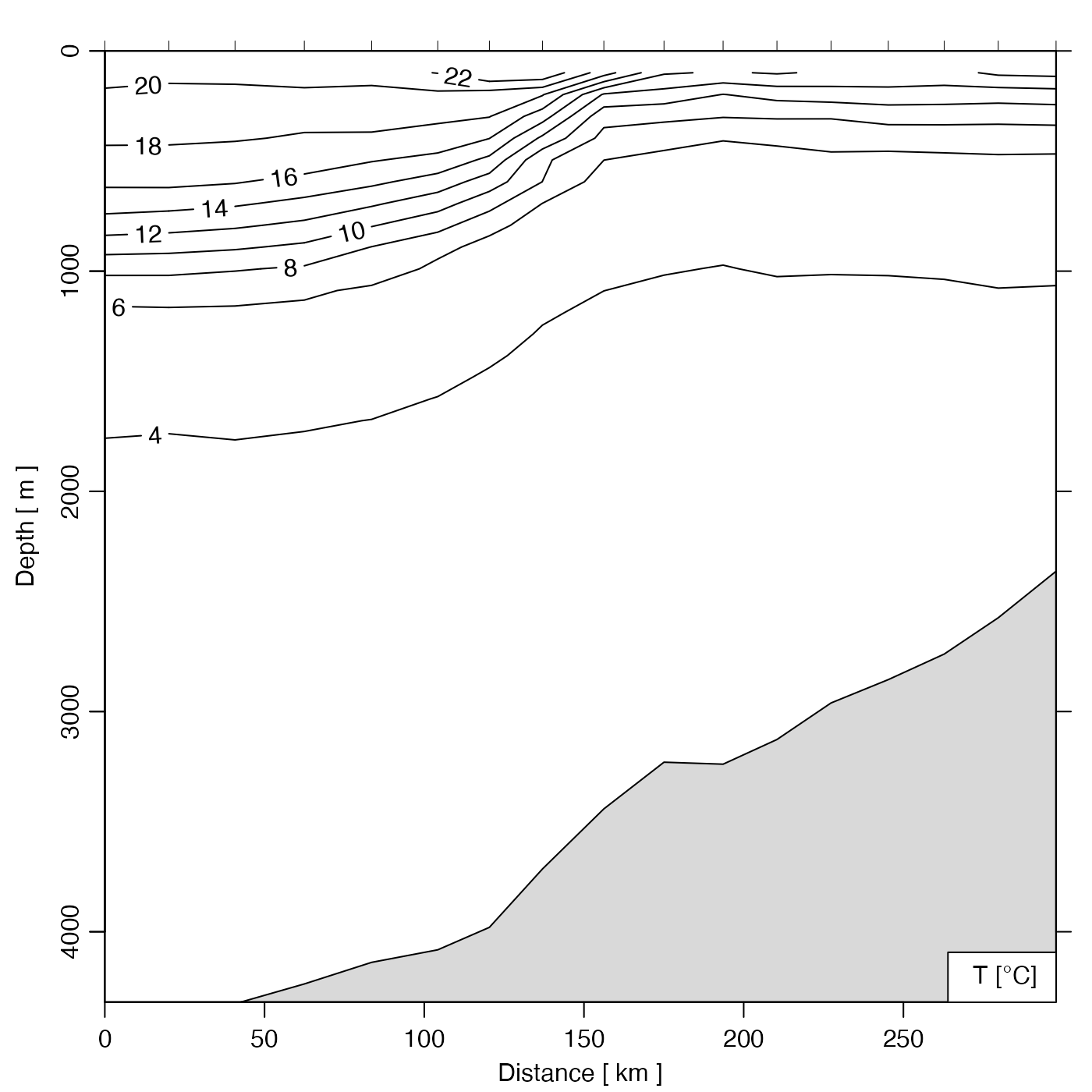Grid a section, by interpolating to fixed pressure levels. The "approx", "boxcar" and "lm" methods are described in the documentation for ctdDecimate(), which is used to do this processing.

sectionGrid(
section,
p,
method = "approx",
trim = TRUE,
debug = getOption("oceDebug"),
...
)

## Arguments

section A section object containing the section to be gridded. Optional indication of the pressure levels to which interpolation should be done. If this is not supplied, the pressure levels will be calculated based on the typical spacing in the ctd profiles stored within section. If p="levitus", then pressures will be set to be those of the Levitus atlas, given by standardDepths(). If p is a single numerical value, it is taken as the number of subdivisions to use in a call to seq() that has range from 0 to the maximum pressure in section. Finally, if a vector numerical values is provided, perhaps. constructed with seq() or standardDepths(5) (as in the examples), then it is used as is, after trimming any values that exceed the maximum pressure in the station data stored within section. The method to use to decimate data within the stations; see ctdDecimate(), which is used for the decimation. Logical value indicating whether to trim gridded pressures to the range of the data in section. an integer specifying whether debugging information is to be printed during the processing. This is a general parameter that is used by many oce functions. Generally, setting debug=0 turns off the printing, while higher values suggest that more information be printed. If one function calls another, it usually reduces the value of debug first, so that a user can often obtain deeper debugging by specifying higher debug values. Optional arguments to be supplied to ctdDecimate(), e.g. rule controls extrapolation beyond the observed pressure range, in the case where method equals "approx".

## Value

A section object that contains stations whose pressure values match identically, and that has all flags set to NA.

## Details

The default "approx" method is best for bottle data, the "boxcar" is best for ctd data, and the "lm" method is probably too slow to recommend for exploratory work, in which it is common to do trials with a variety of "p" values.

The stations in the returned value have flags with names that match those of the corresponding stations in the original section, but the values of these flags are all set to NA. This recognizes that it makes no sense to grid flag values, but that there is merit in initializing a flag system, for possible use in later processing steps.

Other things related to section data: [[,section-method, [[<-,section-method, as.section(), handleFlags,section-method, initializeFlagScheme,section-method, plot,section-method, read.section(), section-class, sectionAddStation(), sectionSmooth(), sectionSort(), section, subset,section-method, summary,section-method

## Examples

# Gulf Stream
library(oce)
data(section)
GS <- subset(section, 109<=stationId&stationId<=129)
GSg <- sectionGrid(GS, p=seq(0, 5000, 100))
plot(GSg, map.xlim=c(-80,-60))# Show effects of various depth schemes
par(mfrow=c(3, 1))
default <- sectionGrid(GS)
approxML <- sectionGrid(GS, method="approxML")
standardDepths5 <- sectionGrid(GS, p=standardDepths(5))
plot(default, which="temperature", ztype="image", ylim=c(200,0))
mtext("default sectionGrid()")
plot(approxML, which="temperature", ztype="image", ylim=c(200,0))
mtext("sectionGrid(..., method=\"approxML\")")
plot(standardDepths5, which="temperature", ztype="image", ylim=c(200,0))mtext("sectionGrid(..., p=standardDepths(5))")